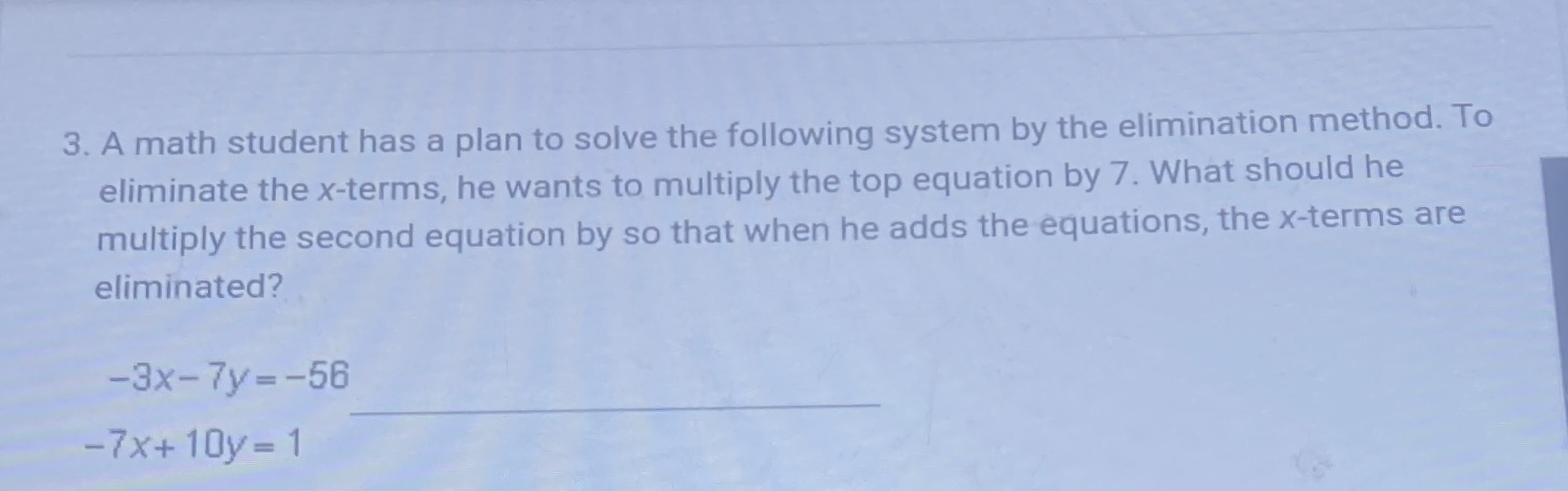### ¿Todavía tienes preguntas de matemáticas?

Pregunte a nuestros tutores expertos
Algebra
Pregunta3. A math student has a plan to solve the following system by the elimination method. To eliminate the $$x$$ -terms, he wants to multiply the top equation by $$7$$ . What should he multiply the second equation by so that when he adds the equations, the $$x$$ -terms are eliminated?

$$- 3 x - 7 y = - 56$$

$$- 7 x + 10 y = 1$$